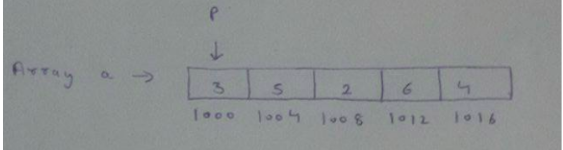# GATE | GATE-CS-2016 (Set 2) | Question 47

Consider the following program:

 `int` `f(``int` `*p, ``int` `n) ` `{ ` `    ``if` `(n <= 1) ``return` `0; ` `    ``else` `return` `max(f(p+1,n-1),p-p); ` `} ` `int` `main() ` `{ ` `    ``int` `a[] = {3,5,2,6,4}; ` `    ``printf``(``"%d"``, f(a,5)); ` `} `

Note: max(x,y) returns the maximum of x and y. The value printed by this program is

(A) 2
(B) 3
(C) 4
(D) 5

Explanation:
Look at the recursion stack of the given code in the below image. Assuming that the base address of array starts from 1000 and an integer takes 4 Bytes.After the last recursive call f(1016,1) returns, in the previous call we will have return max(0,2) and then return max(2,-4) and then return max(2,3) and then finally return max(3,-2) = 3.

This solution is contributed by Pranjul Ahuja

Quiz of this Question

My Personal Notes arrow_drop_up
Article Tags :

1

Please write to us at contribute@geeksforgeeks.org to report any issue with the above content.